ICSESolutions.com provides ICSE Solutions for Class 10 Chemistry Chapter 2 Chemical Bonding for ICSE Board Examinations. We provide step by step Solutions for ICSE Chemistry Class 10 Solutions Pdf. You can download the Class 10 Chemistry ICSE Textbook Solutions with Free PDF download option.

## ICSE Class 10 Chemistry Chapter 2 Chemical Bonding Solutions

### Short Questions

Question 1: The combination of atoms to form molecules is based on octel rule. Give two limiations of this rule.
Answer: (i) It cannot explain the formation of molecules like BeF2, BF3 in which the central atom has less than 8 electrons in its valence shell.
(ii) It cannot explain the formation of molecules such as PF5, SF6 etc., in which central atom has more than eight electrons in its valence shell.

Question 2: Write the general characteristics of electrovalent compounds.
Answer: The general characteristics of electrovalent compounds are:
(i) Electrovalent compounds are mostly crystalline in nature.
(ii) Electrovalent compounds form hard crystals. These crystals are usually brittle.
(iii) Electrovalent compounds have high density with high melting and boiling points.
(iv) Electrovalent compounds are soluble in polar solvents.
(v) Electrovalent compounds exhibit isomorphism.
(vi) They react very fast.

Question 3: On what factors the formation of an electrovalent compound depends ?
Answer: The formation of an ionic compound depends on the following factors:
(i) Low ionisation energy: Lesser is the ionisation energy of an atom, greater will be its tendency to form cation by losing the valence electron. Metals with lower ionisation energy values have a greater tendency to form ionic bonds.
(ii) High electron affinity: Higher the value of electron affinity, greater will be the tendency of the atom to gain electron and form an anion. Elements with high electron affinity values form ionic compounds.
(iii) High lattice energy: The higher is the value of lattice energy, greater will be the electrostatic force of attraction between the oppositely charged ions and hence ionic compounds are formed with greater ease by the release of energy.

Question 4: What are the salient features of electrovalency?
Answer: Some salient features of electrovalency are mentioned below :
(i) An electrovalent bond is formed by loss or gain or transfer of electrons.
(ii) Ions are formed during the formation of an electrovalent bond.
Positive ions —> Cations (Na+, K+, Ca2+, etc.)
Negative ions —> Anions (Cl, O2-, N3-, etc.)
(iii) An electrostatic force of attraction exist between the oppositely charged ions.
(iv) There is one fixed direction in space among the ions.

Question 5: Write important general characteristics of covalent compounds.
Answer: The important general characteristics of covalent compounds are as follows:
(i) Usually covalent compounds exist in gaseous, liquid or amorphous state.
(ii) Covalent compounds have low melting or boiling points.
(iii) Except for graphite, covalent compounds are bad conductors of electricity.
(iv) Covalent compounds are soluble in non polar solvents (usually organic solvents). Polar cgvalent compounds are however soluble in polar solvents.
(v) Covalent compounds contain molecules and they undergo reactions slowly.
(vi) Many covalent compounds exhibit various types of isomerism.

Question 6: (i) Name the charged particles which attract one another to form electrovalent compounds.
(ii) In the formation of electrovalent compounds, electrons are transferred from one element to another. How are electrons involved in the formation of a covalent compound?
(iii) The electronic configuration of nitrogen is 2, 5. How many electrons in the outer shell of a nitrogen atom are not involved in the formation of a nitrogen molecule?
(iv) In the formation of magnesium chloride (by a direct combination between magnesium and chlorine), name the substance that is oxidized and the substance that is reduced.
(ii) There is a mutual sharing of electrons
(iii) 2
(iv) Magnesium is oxidised and chlorine is reduced.

Question 7: In the formation of compound XY2, matrix X gives one electron to each Y atom. What is the nature of bond to XY2? Give four properties of XY2.
Answer: The bond in XY2 is ionic.
Properties:
(i) It is hard and brittle.
(ii) It is soluble in water.
(iii) It has high melting and boiling point.
(iv) It does not conduct electric current in the solid-state but conducts electric current in the molten or dissolved state.

Question 8: There are three elements E, F, G with atomic numbers 19, 8, and 17 respectively.
(i) Classify the elements as metals and non-metals.
(ii) Give the molecular formula of the compound formed between E and G and state the type of chemical bond in this compound.Question 9: (i) Give one property of hydrogen chloride which agrees with it being a covalent compound.
(ii) Give one property of magnesium chloride which agrees with it being an ionic compound.
(iii) Name one compound which is covalent, but on dissolving in water conducts electricity?
(iv) Which property of the above compound agrees with the being of a covalent compound?
Answer: (i) Hydrogen chloride is a gas at room temperature and in a dry state, it is a bad conductor of electricity.
(ii) Magnesium chloride in the molten state or in an aqueous solution is a good conductor of electricity and therefore is an ionic compound.
(iii) Hydrogen chloride.
(iv) Hydrogen chloride is a gas. In dry state, it is bad conductor of electricity. Hence, it is a covalent compound.

Question 10: A compound has the formula H2Y (Y = Non-metal). State the following :
(i) the outer electronic configuration of Y.
(ii) the valency of Y.
(iii) the bonding present in H2Y.
(iv) the formula of the compound formed between calcium $$_{ 20 }^{ 40 }{ Ca }$$ and Y.
Answer: (i) Y has six electrons in its valence shell.
(ii) 2
(iii) Covalent
(iv)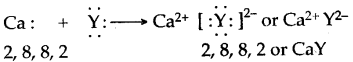Question 11: Elements X, Y, and Z have atomic numbers 6, 9, and 12 respectively. Which are:
(i) forms an anion (ii) forms a cation?
(iii) has four electrons in its valence shell?

 Element Atomic No. Electronic configuration X 6 2,4 Y 9 2,7 Z 12 2,8,2

Answer: (i) Y will form an anion (ii) Z forms a cation
(iii) X has four electrons in its valence shell.

Question 12: (i) Name two compounds that are covalent when taken pure but produce ions when dissolved in water.
(ii) For each compound, give the formulae of the ions formed in aqueous solutions.
(iii) Give the structure of hydroxyl ion.
Answer: (1) Hydrogen chloride and hydrogen bromide.Question 13: How is a co-ordinate bond formed?
Answer: The formation of co-ordinate bond between two atoms to occur in the following two steps:
E.g. two atoms are A and B—in the first step the donor atom A transfers one electron of its lone pair to the acceptor atom B. This result in that atom A develops unit positive charge and atom B develops a unit negative charge. This charge is known as formal charge. This is similar to the formation of ionic bond.
In the second step the two electrons, one each with A and B- are shared by both the ions. This is similar to the formation of covalent bond.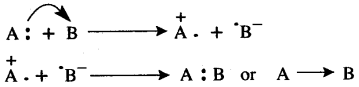Thus, a co-ordinate bond is equivalent to a combination of an electrovalent bond and a covalent bond. Hence it is also called a semipolar bond or dotive bond.

Question 14: What are the characteristics of co-ordinate compounds?
Answer: The general characteristics of co-ordinate compounds are as follows:
(i) Co-ordinate compounds are identical to normal covalent compounds.
(ii) It is rigid and has directional properties.
(iii) These compounds are soluble in non-polar solvents and insoluble in polar solvent.
(iv) These compounds behave as non-conductors of electricity.
(v) The melting and boiling points are higher than covalent compounds and lower than ionic compounds.
(vi) These are stable compounds.

Question 15: Which conditions are necessary for the formation of co-ordinate bond?
Answer: For the formation of a co-ordinate bond the atom acting as a donor must have one unused pair of electrons which may be donated by it to the acceptor atom. The acceptor atom must have an empty orbital to accept the lone pair of electrons.

Question 16: Explain the formation of H3O+ and NH4+ ion.
Answer: Formation of Hydronium ion, H3O+ : This ion formed by the combination of H2O molecule and H+ ion. The water molecule has two O—H covalent bonds and central oxygen atom has two lone pairs of electrons. H+ ion has one vacant ls-orbital. During the formation of H3O+, one pair of lone pair from O-atom is donated to the vacant ls-orbital of H+ ion and O — H co-ordinate bond is formed. Thus H3O+ ion contains two O—H covalent bonds and one O— H co-ordinate bond. After the formation O→ H+ co-ordinate bond becomes identical to the two O—H covalent bonds.
Hence in H3O+ ion all the three bonds are identical.Formation of NH4+, Ammonium ion : This ion formed by the combination of NH3 molecule and H+ ion. In NH3 molecule each of three H-atoms is linked to N-atom by a covalent bond. Thus in this molecule, N-atom is left with a lone pair of electrons after completing its. octet by sharing three of its valence shell electrons with three H-atoms. The electrons of lone pair on N-atom are donated to H+ ion and thus a N/EH co-ordinate bond is established in NH4+ ion.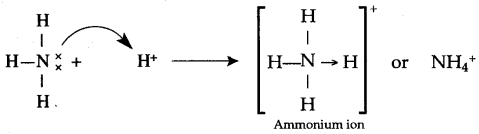Question 17: What is the lone pair effect? In what kind of compound does this effect occur?
Answer: When the unshared pair of electrons around an atom in the middle of a molecule is completely shared by another atom or an ion, it is called the lone pair effect. The lone pair effect is shown by polar covalent compounds such as HCl and NH3.

Question 18: (i) Which of the following is not a common characteristic of an electrovalent compound?
(a) High melting point
(b) Conducts electricity when motten
(c) Consists of oppositely charged ions
(d) Ionises when dissolved in water
(ii) What are the terms defined below:
(a) A bond formed by a shared pair of electrons with both electrons coming from the same atom.
(b) A bond formed by a shared pair of electrons, each bonding atom contributing one electron to the pair.
(ii) (a) Co-ordinate bond. (b) Covalent bond.

Question 19: (i) Acids dissolve in water to produce positively charged ions. Draw the structure of these positive ions.
(ii) Explain why Carbon tetrachloride does not dissolve in water.
(iii) Elements Q and S react together to form an ionic compound. Under normal conditions, which physical state will the compound QS exist in?(ii) Because carbon tetrachloride is a non-polar covalent compound whereas water is a polar covalent solvent.
(iii) Solid-state.
(iv) No. Because to form an ionic compound if one element gives electrons, the other element should accept electrons. Metals can only lose electrons to provide +ve ions.

Question 20: Predict the type of bonding in the following molecules :
(i) Oxygen (ii) Calcium oxide
(iii) Water (iv) Methane
(v) Ammonium ion (vi) Nitrogen
(vii) Magnesium chloride (viii) Carbon dioxide
(ix) Carbon tetra chloride (xi) Hydrogen cyanide (x) Hydrogen chloride
Answer: (i) Covalent bond (ii) Ionic bond
(iii) Covalent bond (iv) Covalent bond
(v) Covalent bond (vi) Ionic bond
(vii) Covalent bond (viii) Covalent bond
(ix) Covalent bond (xi) Covalent and co-ordinate bonds (x) Covalent bond

### Figure/Table Based Questions

Question 1: With the help of the figure, explain that the methane molecule is a non-polar covalent compound?
Answer: A covalent bond between two atoms is non-polar if the electrons involved in bond formation are equally shared between two atoms having similar electronegativities. For example, in the case of the methane molecule, the four-electron pairs shared between one carbon atom and four hydrogen atoms lie exactly in between one carbon atom and four hydrogen atoms and hence form non-polar bonds.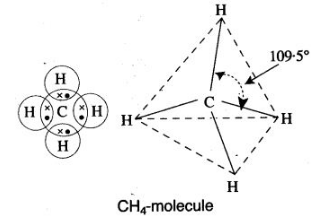The three-dimensional structure of the methane molecule has the carbon atom at the centre of the tetrahedron and four hydrogen atoms are located at the corners of the tetrahedron. In such a configuration, none of the participating atoms is more electrically charged. So, methane is a non-polar covalent compound.
Electro-negativity of carbon = 2.5 .
Electro-negativity of hydrogen = 2.1

Question 2: With the help of the figure Show HCl is-a polar molecule.
Answer: In HCl molecule the strong nuclear charge of chlorine atom attracts the electron of hydrogen far away from its nucleus, with the result the hydrogen atom develops a slight positive charge (d+) and chlorine atom develops a slight negative charge (d)Question 3: Explain how polar covalent compound HCl which is a bad conductor in pure and liquid state, ionises in water.
Answer: A molecule of HCl is a polar covalent, i.e., the electron pair shared between hydrogen and chlorine is far away from hydrogen than chlorine, with the result that the hydrogen atom has a slight positive charge on it and the atom of chlorine has slight negative charge on it as,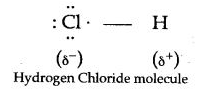When hydrogen chloride is added to water, then water molecules bombard it from all directions. If the alignment of a water molecule is such that a slightly negative oxygen atom faces the slightly positive hydrogen atom of HCl then, the slightly positive hydrogen atom is swallowed by a water molecule in the form of a single proton to form a hydronium ion (H+3O). The residual chlorine atom takes away the electron of hydrogen atom along with it so as to form chloride ion as illustrated below: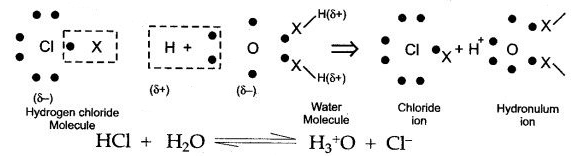Question 4: How does common salt solution ionize in water which is a polar covalent compound?
Answer: When the sodium chloride crystals come in contact with water, they are bombarded by water molecules from all possible directions.
When the molecules of water bombard the sodium ion, such that the slightly negatively charged oxygen atom faces sodium ion, then they exert an electrostatic pull on it. If a sufficient number of water molecules strike a particular sodium ion in the same fashion, then it can be pulled out of the crystal of sodium chloride to form a free sodium ion.Similarly, if the molecules of water bombard the chloride ion, such that the slightly positively charged hydrogen atoms face chloride ion then they exert an electrostatic pull on it. If a sufficient number of water molecules strike a particular chloride ion in the same fashion, then it can be pulled out of the crystal of sodium chloride to form a free chloride ion.
Figures (b) and (c) show a sodium ion and a chloride ion has been pulled out of the crystalline structure by water molecules. However, it is not actually known that how many molecules of water are necessary to pull out a particular ion from its crystal.

Question 5: Draw dot diagrams to illustrate the structure of the molecules of:
(i) Ammonia (ii) Carbon dioxide
(iii) Methane (iv) Water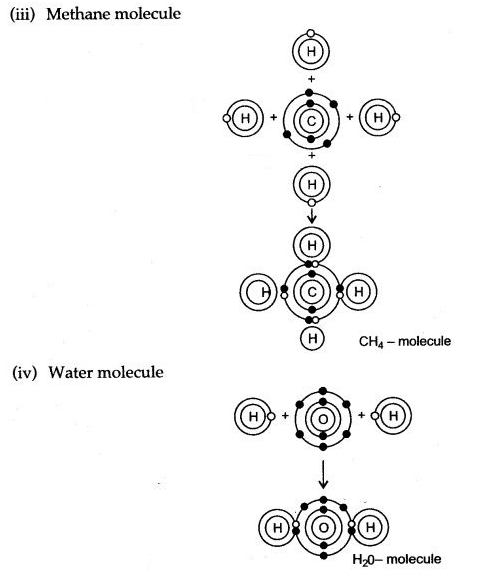Question 6: Give the electron dot structures of:
(i) NaCl (ii) MgCl2 (iii) CaO (iv) Cl2 (v) H2O (iv) NH3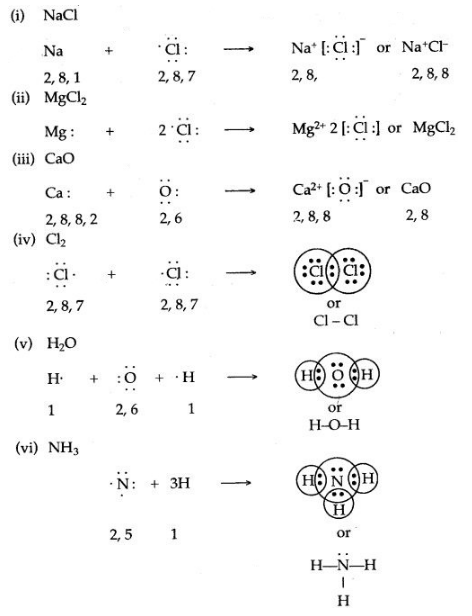Question 7: Draw an electron dot diagram to show the structure of hydronium ion. State the type of bonding present in it.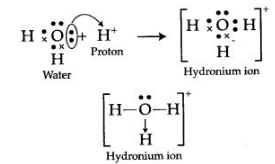The type of bonding present in hydronium ion is co-ordinate bonding.

Question 8: By drawing an electron dot diagram, show the lone pair effect leading to the formation of ammonium ion from ammonia gas and hydrogen ion.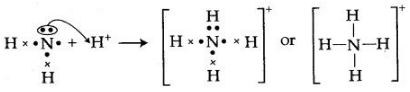NH3 has one lone pair of electrons which is donated to a hydrogen atom forming a co-ordinate bond. The arrow represents a co-ordinate bond. The arrow points from donor to acceptor atom.

### Reasoning Based Questions

Question 1: Why atoms combine with one another?
Answer: The cause of chemical combination between atoms of the various elements is their tendency to acquire nearest stable noble gas configuration of the octet of electrons and duplet of electrons in case of hydrogen atoms in their outermost shells.

Question 2: Why do certain elements form ions?
Answer: Every particle (molecule, atom, or ion) has the tendency to attain the state of lowest energy. As atoms of all elements except the noble gases, have one to seven electrons in their outermost shell, therefore, they are not in the state of minimum energy. They are reactive. In order to attain a minimum state of energy, i.e., to acquire a duplet or octet structure in their valence shell, they either donate or accept electrons. In this process, they become charged particles or ions.

Question 3: Why are all atoms other than noble gas atoms, reactive?
Answer: Because they are short of octet in their outermost shell. They tend to attain eight electrons in their outermost shell to acquire nearest inert gas electronic configuration.

Question 4: Why a molecule of hydrogen is more stable than uncombined atoms?
Answer: When a molecule of hydrogen is formed from the atoms, energy is released (104 kcal/ mol). The molecules possessing lower energy are more stable, than the atoms. Hence molecule of hydrogen is more stable than uncombined atoms.

Question 5: Why is hydrogen ion called proton?
Answer: An atom of hydrogen has one proton in its nucleus and one electron in its valence shell. It donates its valence electron, the residual ion consists of a single proton. It is on account of this fact, that hydrogen ion is called proton.

Question 6: A cation is smaller than the atom from which it is formed. Why ?
Answer: In the formation of a cation, the atom loses the electrons of its outer shell. Thus, the cation has one electron shell less than the atom, from which it is formed. So, it is smaller than the atom.

Question 7: An anion carries negative charge. Why ?
Answer: When an atom forms an anion, it gets one or more electrons (from another atom) in its outer shell. As the number of electrons in the outer shell is increased, the repulsion between them increases. This makes the shell expand outward. Further, since the number of protons remains the same as in the neutral atom, the nucleus attracts the increased number of electrons less strongly. For these reasons the anion is bigger than the parent atom.

Question 8: Why electrovalent compounds form hard crystals ?
Answer: The crystals of electrovalent compounds are made up of crystal lattice containing oppositely charged ions. Each cation is surrounded by a definite number of anions and vice-versa. Their is a great electrostatic force of attraction among these oppositely charged ions and as a result, ionic compounds form hard crystals.

Question 9: Why electrovalent compounds have high melting, boiling points and low volatility ?
Answer: There is a strong force of attraction among the oppositely charged ions in the crystals of electrovalent compounds. Therefore, a large amount of energy is required to separate them. Due to these strong forces of attraction, ionic compounds have high melting and boiling points and low volatility.

Question 10: Why electrovalent compounds in crystalline state do not conduct electricity ?
Answer: Electrovalent crystalline solids do not conduct electricity because the oppositely charged ions in them are held together by a strong electrostatic force of attraction. These ions occupy fixed position in the crystals and do not move when an electric field is applied. .

Question 11: Why do electrovalent compounds usually dissolve in water and molecular compounds dissolve in organic solvents ?
Answer: Water is polar molecule.%It hydrates ions by its polar attraction and pulls the ions apart. In covalent compounds the polar charge on molecules is weak and does not have that much of attractive force on the molecules, organic solvents exert a greater polar attraction on covalent molecules and hence dissolve them.

Question 12: Why ionic compounds are generally soluble in water, but insoluble in organic solvents ?
Answer: The water molecules have high dielectric constant thus, water molecules easily break the ionic bonds between the ions. The ions drift in water in all possible directions and hence, ionic compounds dissolve in water, while organic solvents are non-polar in nature and hence, cannot break the ionic bonds. Thus, the ionic compounds do not dissolve in them.

Question 13: Why molten NaCl conduct electricity but, CCl4 does not ?
Answer: Molten NaCl contains Na+ and Cl ions, which are free to move, hence it conducts electricity. Whereas Liquid CCl4 does not contain any charged particles to conduct electricity.

Question 14: Why is sodium ion (Na+) not reactive, but sodium metal is very reactive ?
Answer: Sodium ion has eight electrons in its valency shell and it is the minimum state of energy, while the sodium metal has one electron in valency shell, so the sodium metal is very reactive.

Question 15: Why covalent compounds are generally liquids or gases ?
Answer: In covalent compounds, the molecules are held together by weak Van der Waal’s forces. In liquids, the molecules are weakly attracted whereas in gases, these forces are almost non¬existent. Hence, they are generally liquids or gases.

Question 16: Why all covalent compounds are bad conductor of electricity ?
Answer: The covalent compounds do not have positive or negative ions in their fused state. Thus, when electric potential is supplied, no ions migrate to opposite poles and hence no conduction of electric current takes place.

Question 17: Why covalent compounds have low melting point and boiling point ?
Answer: The force of attraction between the molecules is very weak and so the amount of energy needed to separate them is small, consequently they have low melting points and boiling points.

Question 18: Why most of the covalent compounds have density less than that of water ?
Answer: The covalent molecules are held very weakly by van der Waal’s forces. Thus, there are large inter molecular spaces between the molecules. In other words the number of molecules per unit volume is less. Thus mass per unit is also less and hence, covalent compound have low density.

Question 19: Why do covalent compounds exist as gases, liquids or soft solids ?
Answer: Covalent compounds exists as gases, liquids or soft solids because they have weak forces of attraction between their molecules.

Question 20: Why hydrogen chloride can be termed as a polar covalent compound ?
Answer: Pure covalent bond exists between two elements which have similar electronegativities. In hydrogen chloride, chlorine being more electronegative attracts the shared pair of electrons towards itself. As a result hydrogen acquires partial positive charge and chlorine gets partial r negative charge. Thus, hydrogen chloride can be termed as a polar covalent compound.

Question 21: Why is methane molecule regarded as a non-polar covalent compound ?
Answer: It has been found that a methane molecule has a three dimensional tetrahedral structure. The four carbon hydrogen tetrahedral structure. The four carbon hydrogen bonds are directed towards the four corners of tetrahedron. In such a configuration, none of the participating atoms is more electrically charged as compared to other atoms. Hence methane molecule is a non polar covalent compound.

Question 22: Why the melting and boiling points of co-ordinate compounds are higher than covalent compounds and lower than ionic compounds ?
Answer: A co-ordinate bond is a union of one electrovalent and one covalent bond, the volatility of these compounds lies between that of covalent and ionic compounds. Thus their melting and boiling points are higher than covalent compounds and lower than ionic compounds.

For More Resources